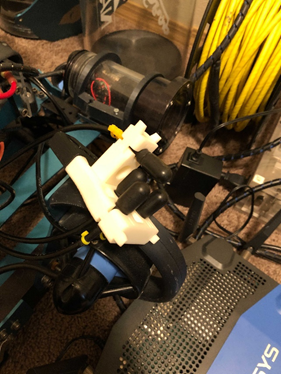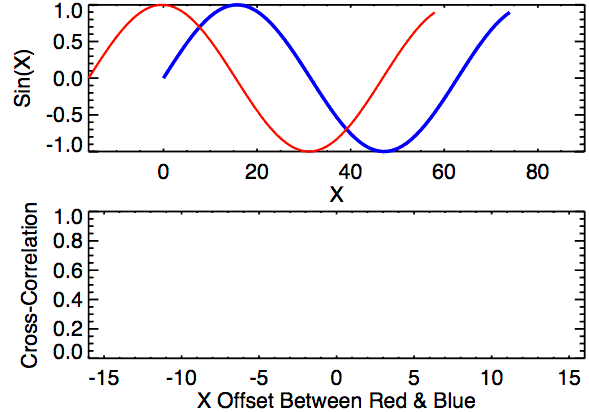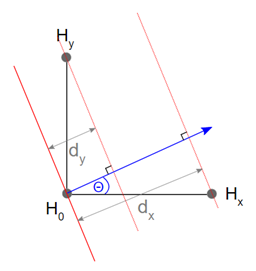# A General Explanation of our Sonar System

Written by Chris Nathman. Posted in General

The last two tasks of the Robosub competition each year can be located by acoustic pingers which are setup near them. Locating the tasks by using these pingers requires a sonar system capable of determining the direction that a ping is coming from. Our sonar system accomplishes this by finding the time delays of the ping’s arrival at 4 hydrophones.Our hydrophones

To get the time delays from the hydrophones, we first wait until the sound level reaches some threshold which indicates we are hearing a ping. We then perform cross correlations between the signal on channel 0 and the signals on the other channels. We shift the signal of each channel other than 0 over by a small amount of time and take the cross correlations between channel 0 again. This is repeated several times and then we take the shift value (a unit of time) of the max cross correlation for each channel as the time delay on that axis.Cross correlating two sine functions at different offsets.

Once the time delays have been found, we can use trigonometry to determine what direction the sound came from. The image below shows how this is done in 2 dimensions, but the same idea applies to 3 dimensions.Finding the ping angle from the time delays. Each dot labeled with an H represents a hydrophone. The red lines represent a sound wave at different times. The blue arrow represents the direction the sound came from.

Notice in the image that the angle θ is part of a right triangle. The hypotenuse of this triangle is the distance from one hydrophone to the other, and one side length is the time delay between those hydrophones multiplied by the speed of sound. Thus θ, which is the direction of the ping, can be found by taking the arccosine of that side length over the hypotenuse. Note that there are multiple angles which can have this cosine so we must also calculate the angle 90 – θ using the same method but with the other hydrophone (Hy). Knowing both θ and 90 – θ tells us what angle the ping came from so we can now use that information to navigate to the tasks.

Bookmaker review site http://wbetting.co.uk/ for William Hill